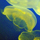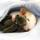## General Question#### Can you figure out how this math trick works?

Asked by LostInParadise (29007) November 5th, 2010
4 responses
“Great Question” (2)

We start with the numbers from 1 to 5. Take any two of them, which we will call a and b. Replace a and b with ab+a+b. If you chose 2 and 4, that would give 2*4+2+4= 14, so we have left:
14 1 3 5. Choose another two numbers and continue this process until there is one number left.

Using my powers of concentration, I can tell you in advance that the number you end up with is 719. What this means, of course, is that the order in which the numbers are chosen does not matter. The question is why? Can you come up with a simple formula for the final number?

There is nothing special about the numbers 1 to 5. If we had chosen any group of numbers, the final answer would be independent of the order in which the numbers are chosen.Follow this questionSend to a friend!Topics: ,
Observing members: 0Composing members: 0Let i originally equal 1.

n
∑ ab+a+b = 719 //719 for this problem, anyway.
i = 1

Where a ≠ b, 1 ≤ a ≤ 5, 1 ≤ b ≤ 5, and b comes one term after a in the sequence. //Technically, order doesn’t matter, but it makes the ∑ make sense.
ab+a+b = z
Replace a and b with the new number z in the sequence and continue the summation.
i = z

So, after the first iteration, the equation becomes:

n
∑ ab+a+b = 719
i = z

1 2 3 4 5
(1*2+1+2) = 5
5 3 4 5

5*3+5+3 = 23

23 4 5

23*4+23+4 = 119

119 5
119*5+119+5 = 719

I would have to pull out my Discrete Mathematics notebook to come up with a simple formula. I’ll come back to this question when I have time. If you’re into Computer Science, think of it as a “for loop.”

As far as why order doesn’t matter, that’s kind of a trick question. The order of operations matters, so that’s why the order of the numbers from a group that you act upon doesn’t; sort of like Commutative Laws, eventually, you would get the same result no matter what two numbers you multiply and add up first in a list.

Auxilium (52)“Great Answer” (6)Let a#b = ab + a + b..
Then (a#b)#c = (a#b)c + a#b + c
...................... = (ab + a + b)c + (ab + a + b) + c
...................... = (ab)c + ac + bc + ab + a + b + c
...................... = a(bc) + ab + ac + a + bc + b + c
...................... = a(bc + b + c) + a + (bc + b + c)
...................... = a(b#c) + a + b#c
...................... = a#(b#c).
Since a#b = b#a, it matters neither which pair is chosen first nor in which order the operation is applied to the chosen pair.

ratboy (15167)“Great Answer” (8)@ratboy, I like your thinking on this.

There is, however, an additional insight that leads to a general equation. The key observation is that ab+a+b = (a+1)(b+1)-1

Using the # notation, see how this works out.
(a#b)#c =[ (a+1)(b+1)-1+1](c+1) – 1 = (a+1)(b+1)(c+1) – 1. In general, for n terms, no matter what order they are combined, you get (a1+1)(a2+1)…(an+1) – 1
For the numbers from 1 to 5, this works out to 2*3*4*5*6 -1 = 719

The mathematical concept of isomorphism provides an elegant way of stating this.

Let T(x)=x+1 Then T(a#b) = T(a)*T(b). The # operation is isomorphic to multiplication. This means that the two operators are structurally equivalent, so commutativity and associativity for # follow from the corresponding properties of multiplication. This also leads to the general formula of
T(a1#a2#...#an) = T(a1)*T(a2)*…*T(an)

LostInParadise (29007)“Great Answer” (2)Let N = {0, 1, 2, ... }, then (N, #) is a commutative monoid since 0#n = n#0 = n for each n.

ratboy (15167)“Great Answer” (0)